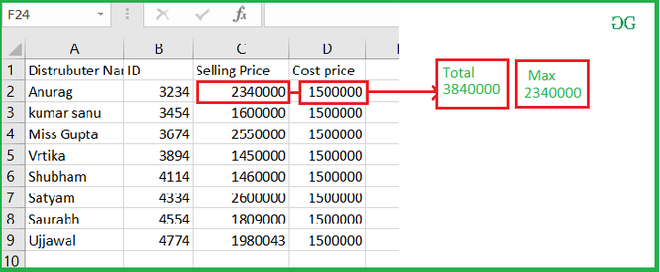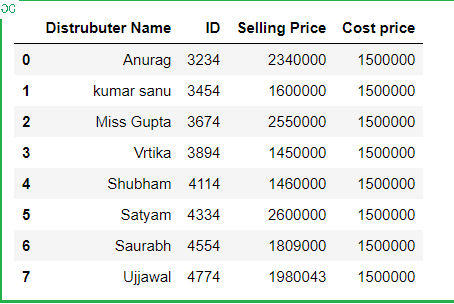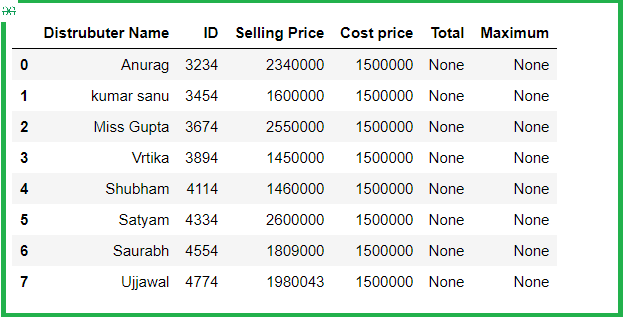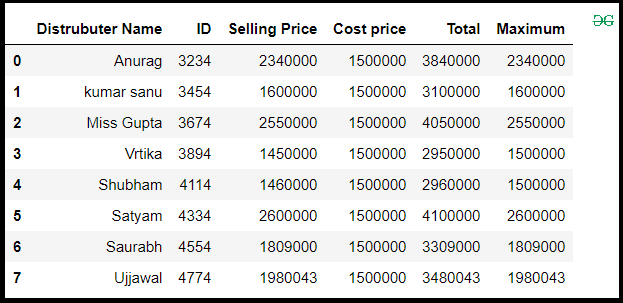Open in App
Not now

# Find the sum and maximum value of the two column in excel file using Pandas

• Last Updated : 27 Aug, 2021

In these articles, we will discuss how to read data from excel and perform some mathematical operation and store it into a new column in DataFrame. Suppose our excel file looks like this.sample_data.xlsx

Then we have to compute the sum of two-column and find out the maximum value and store into a new DataFrame column.

Approach :

• Import Pandas module.
• Create a new column for storing Sum and maximum.
• Set the Index of each column for accessing the element.
• Store the sum of two-columns in a new column.
• And store Maximum number from two columns in a column.
• Display DataFrame.

Step 1: Importing module and reading from excel.

## Python3

 `# import module``import` `pandas as pd` `# read from excel``# and store in a DataFrame``df ``=` `pd.read_excel(``'excel_work/book_sample.xlsx'``)``df`

Output :Step 2: Create a new column for storing sum and max

## Python3

 `# creation new column``df[``'Total'``] ``=` `None``df[``'Maximum'``] ``=` `None``df`

Output :Step 3: Set an index for accessing the required column.

## Python3

 `# Set index for each column``index_selling``=``df.columns.get_loc(``'Selling Price'``)``index_cost``=``df.columns.get_loc(``'Cost price'``)``index_total``=``df.columns.get_loc(``'Total'``)``index_max``=``df.columns.get_loc(``'Maximum'``)` `print``(index_selling,index_cost,index_total,index_max)`

Output :

`2 3 4 5`

Step 4: Select each row and add a column and find maximum

## Python3

 `for` `row ``in` `range``(``0``, ``len``(df)):``    ``df.iat[row, index_total] ``=` `df.iat[row,``                                      ``index_selling] ``+` `df.iat[row, index_cost]``    ` `    ``if` `df.iat[row, index_selling] > df.iat[row, index_cost]:``        ``df.iat[row, index_max] ``=` `df.iat[row, index_selling]``    ``else``:``        ``df.iat[row, index_max] ``=` `df.iat[row, index_cost]``df`

Output :My Personal Notes arrow_drop_up